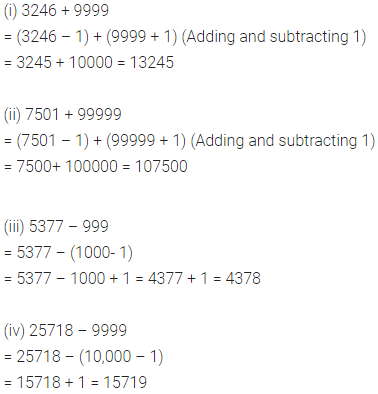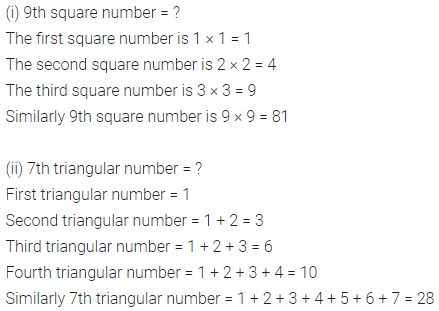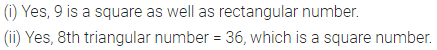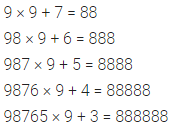# ML Aggarwal Class 6 Solutions for ICSE Maths Chapter 2 Whole Numbers Ex 2.3

## ML Aggarwal Class 6 Solutions for ICSE Maths Chapter 2 Whole Numbers Ex 2.3

Question 1.
Using shorter method, find
(i) 3246 + 9999
(ii) 7501 + 99999
(iii) 5377 – 999
(iv) 25718 – 9999
(v) 123 × 999
(vi) 203 × 9999
Solution:Question 2.
Without using a diagram, find
(i) 9th square number
(ii) 7th triangular number
Solution:Question 3.
(i) Can a rectangular number be a square number?
(ii) Can a triangular number be a square number?
Solution:Question 4.
Observe the following pattern and fill in the blanks:
1 × 9 + 1 = 10
12 × 9 + 2= 110
123 × 9 + 3 = 1110
1234 × 9 + 4 = ……….
12345 × 9 + 5 = …………..
Solution:Question 5.
Observe the following pattern and fill in the blanks:
9 × 9 + 7 = 88
98 × 9 + 6 = 888
987 × 9 + 5 = 8888
9876 x 9 + 4 = …………
98765 × 9 + 3 = ……….
Solution: